The high power amplifier is an important building block in wireless communication systems, but is inherently nonlinear. The nonlinearity results in intermodulation of the input signals, causing intermodulation distortion products at the output of the HPA and hence adjacent channel interference.1 Thus, linearization methods are usually employed in base station HPAs of wireless communications systems to minimize the IMDPs. Many analog predistortion methods have been reported in recent years.2-12 Analog predistortion (APD), with the merits of simple structure and acceptable linearity improvement, is one of the popular linearization techniques used. Generally, in analog predistortion, a predistorted signal (which could be the difference-frequency signals, the harmonic-frequency signals and the in-band intermodulation (IM) signals of the fundamental signals) is generated and then, together with the fundamental signal, fed to the HPA to reduce the IMDPs at the output.6-12 The difference-frequency and harmonic-frequency signals are at much lower and higher frequencies, respectively, from the fundamental signal and so are likely to be blocked by the limited bandwidths of the HPAs. Thus, the method using the in-band IM signals for suppressing the IMDPs is more practical and realistic.

In this article, the design of a fifth-order analog predistorter is proposed, which employs the in-band IM signals for the predistortion of the HPA. Two IM signal generators with identical configuration are designed to generate the third- and fifth-order intermodulation (IM3 and IM5) signals. In the previous design of a third-order analog predistorter,8,9 the IM3 signal and fundamental signal had to be adjusted together, making the tuning process to suppress the IMDP3 very difficult to do. In this proposed design, the IM3 and IM5 signals are generated individually and can be adjusted independently to suppress the IMDP3 and IMDP5, respectively, making the tuning process much easier to do. The fifth-order analog predistorter is designed by computer simulation and studied using a two-tone and π/4-DQPSK signals centered at 2.2 GHz in a practical base station 100 W HPA, HPA2100-085-SW01, manufactured by Bravotech Inc., China, for use in cellular mobile systems. The final design of the predistorter is implemented and the performance is measured for verification. The simulation and measured results show very good agreement, and excellent performance on predistortion of the HPA at an output power of 65.5 W.

### Theory and Configuration of the Predistorter

Predistortion Principle
The nonlinear characteristic of a HPA can be represented in the form of a power series expression:1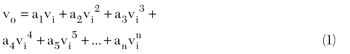where vo and vi are the output and input signals, respectively, and a1, ..., an are the coefficients used to curve-fit the actual HPA characteristic. Consider an input signal consisting of two tones with equal amplitude and small frequency spacing:The output signal from the HPA is:Each of the terms, except the first-order term, in Equation 3 will generate a number of distortion products. For example, the second-order term generates the second-order distortion products at frequencies 2ω1, 2ω2 and ω1±ω2; the third-order term generates the third-order distortion products at frequencies ω1, ω2, 3ω1, 3ω2, 2ω1±ω2 and 2ω2±ω1. It can be shown that the even-order terms in Equation 3 generate the distortion products that are out of band, so the distortion products of most concern are the ones close to the two-tone signal, such as the IMDP3 at frequencies 2ω12 and 2ω21 generated in the third-order term of Equation 3.Figure 1 Block diagram of the fifth-order distortion using in-band IM signals.

Figure 1 shows the basic principle of the proposed fifth-order predistorter. To simplify the description, assume the input signal, vin, to the predistorter is a two-tone signal and the HPA can be modeled using only the first three odd-number terms in Equation 3. Assume that the IM3 and IM5 signals are generated and add them to the two-tone signal in the predistorter to produce an HPA input signal vp: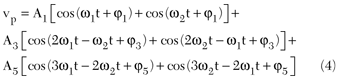where A1, A3, A5 and φ1, φ3, φ5 are the amplitudes and phases of the fundamental (two-tone) signals, IM3 and IM5 signals, respectively. The amplitudes and phases of the IM3 and IM5 signals are adjustable in the predistorter. The output signal from the HPA is:Expansions of the third- and fifth-order terms of Equation 5 are very difficult and complicated. To simplify the expansions, consider only the IMDP3 and IMDP5, which have the most power. It can be readily shown that the IMDP3 and IMDP5, respectively, are given by:Note that, in general, adjacent coefficients in the odd-number terms of Equation 1 have opposite signs.7 Taking this into account and setting VIMDP3 = 0 in Equation 6, the condition for cancelling off the IMDP3 is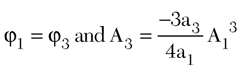which can be achieved by adjusting the signals in Equation 4. Under this condition, the IMDP5 of Equation 7 becomes: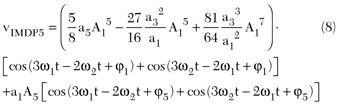which indicates that the IMDP5 can also be cancelled off by adjusting the magnitude A5 and phase φ5 of the input IM5 signal in Equation 4.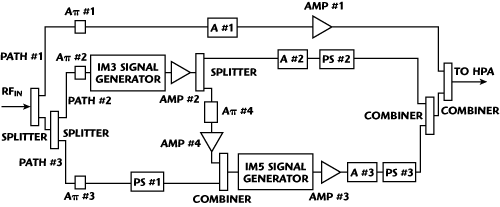Figure 2 Block diagram of the fifth-order predistorter.

The Fifth-order Predistorter
Figure 2 shows the block diagram of the proposed fifth-order predistorter. Three π-resistive attenuators Aπ #1, Aπ #2 and Aπ #3 are used to control the power levels of the input signals in Path #1, Path #2 and Path #3. Among these three paths, Path #1 has a much higher signal power to the HPA and so the voltage-variable-attenuator A #1, together with the linear low power amplifier Amp #1 in Path #1, are used to determine the HPA operating point. The input signal in Path #2 is fed to the IM3 signal generator to generate the IM3 signal, which is then amplified by Amp #2 and split into two paths. One of the IM3 signals is adjusted in amplitude and phase using the voltage-variable attenuator A #2 and the phase shifter PS #2, respectively, for predistortion. The other IM3 signal is adjusted by Aπ #4 and Amp #4 to obtain a proper power level, combined with the input signal in Path #3, which is adjusted by Aπ #3 and PS #1 and then fed to the IM5 signal generator to produce the IM5 signal. The amplitude of the IM5 signal is adjusted using Amp #3 and A #3 and the phase is adjusted using PS #3. The resultant signals in Paths #1, #2 and #3 are combined together and fed to the HPA for suppression of the IMDP3 and IMDP5 at the HPA output.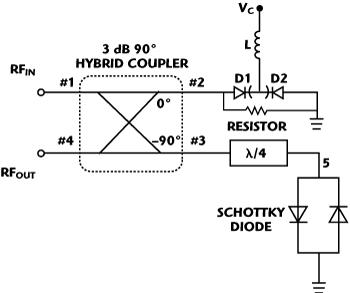Figure 3 Block diagram of the IM signal generator.

The configuration of the IM3 and IM5 signal generators used in the predistorter is shown in Figure 3. It consists of a 3 dB, 90° hybrid coupler, a pair of anti-parallel Schottky diodes, a λ/4-length transmission line at the frequency of 2.2 GHz, two varactors (D1 and D2), an RF choke inductor L and a resistor. Ports #1 and #4 of the 3 dB, 90° hybrid coupler are used as the input and output ports, RFin and RFout, respectively, of the generator. The DC voltage Vc applied to D1 and D2 through the RF choke inductor L is used to control their reactances and hence the phase shift of the reflected signal. The anti-parallel Schottky diodes circuit is basically a mixer with its current flow given by:1Where V(t) is the signal across the anti-parallel Schottky diodes, k is a constant and Is is the saturation current of the diodes.

In Equation 9, at low signal level of V(t), the first- and third-order terms are dominant and other higher-order terms can be neglected. Then the current can be approximated by: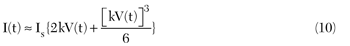IM3 Generation
Let the input signal to the IM signal generator of Figure 3 be a two-tone signal with same magnitude and a small frequency separation:This input signal is split equally into Ports #2 and #3 of the coupler. The signal from port #3 is intermodulated in the anti-parallel Schottky diode circuit through Equation 10 and produces a mixing product consisting of the IM3 signal and the fundamental signal. This mixing product is reflected to Port #4, where the fundamental signal in port #2 is also reflected into the coupler. By appropriately adjusting the resistor and the DC voltage Vc at port #4, the fundamental signal reflected from port #2 can have equal amplitude but 180° phase difference with the fundamental signal reflected from the anti-parallel Schottky diode circuit, thus cancelling each other at the Port #4 and leaving only a strong IM3 signal.

IM5 Generation
The same configuration in Figure 3 can be used as an IM5 signal generator. Here, the IM3 signals generated from the IM3 generator is combined with the fundamental signal to produce an input signal:At port #3 of the coupler, the signal across the anti-parallel Schottky-diode circuit has only half of the power in Equation 12 and can be written as:Substituting Equation 13 into Equation 10 yields the mixing product consisting of the fundamental signal, IM3, IM5, IM7 and IM9 signals given, respectively, by: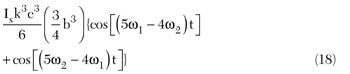From Equations 16 to 18, it can be seen that, if a is much larger than b, say 5 dB, the IM5 signal in Equation 16 will be much larger than the IM7 signal and the IM9 signal, which therefore can be neglected. Same as in the IM3 signal generator, where the resistor and the DC voltage Vc can be adjusted to cancel either the fundamental in Equation 14 or the IM3 signal in Equation 15 at port RFout. Note that both the fundamental and IM3 signals cannot be cancelled simultaneously because these two conditions have different requirements to satisfy. In this design, the IM3 signal is chosen to be cancelled, leaving the fundamental signal uncancelled at port #4. In fact, as explained here, there is no need to cancel the fundamental signal, because the output signal from the IM5 generator is eventually combined with a much stronger fundamental signal from Path #1 (see Figure 2) and then fed to the HPA for predistortion. Thus, as far as the design is concerned, the generator in Figure 3 can be used as an IM5 signal generator.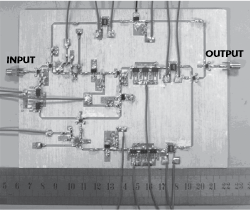Figure 4 Photograph of the fifth-order predistorter.

### Experimental Results

The fifth-order predistorter of Figure 2 has been studied and designed using the computer simulation tool ADS2009 and the final design has been implemented on a Roger's RO4005C substrate with an area of 16×12 cm, as shown in Figure 4. In the implementation, the linear low power amplifiers Amp #1 to Amp #4 used are RFMD's SBB5089, the attenuators A #1 to A #3 are Skyworks' AV103, the phase shifters PS #1 to PS #3 are Skyworks' PS214-315, the two-way splitters and combiners are Skyworks' PD-22 and the resistors in the π-resistive attenuators Aπ #1 to Aπ #4 are 0603 mounting resistors. The IM3 and IM5 signal generators have the same configuration as shown in Figure 3, where the 3 dB, 90° hybrid couplers are Anaren's JP503, the Schottky diodes are Avago's HSMS 282X, the varactors are Skyworks' SMV1245-011, and the resistors and inductor are 0603 mounting components.Figure 5 Output signal spectra from the IM3 signal generator: (a) simulated and (b) measured.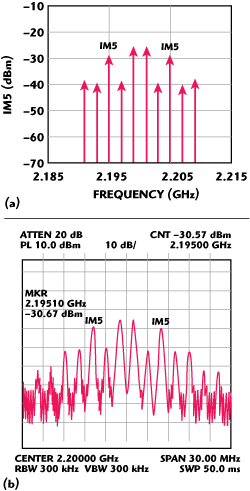Figure 6 Output signal spectra from the IM5 signal generator: (a) simulated and (b) measured.

To study the performances of the IM3 and IM5 signal generators, a two-tone signal, centered at 2.2 GHz and having a 2 MHz spacing has been used as the input signal. The simulated and measured results of the IM3 and IM5 generator are shown in Figures 5 and 6, respectively. It can be seen that the simulated and measured results agree very well. Figure 5 shows that the IM3 signal is more than 20 dB larger than the fundamental signal and the other IM signals. Figure 6 shows that the IM5 signal is more than 10 dB larger than the other IM signals and slightly smaller than the fundamental signal for the reason described previously. However, measurement results have shown that, in normal operation of the predistorter, the required level for the fundamental signal in the main path (that is Path #1 of Figure 2) is more than 20 dB higher than that of the fundamental signal from the IM5 generator, thus the fundamental signal generated by the IM5 generator can be neglected and need not be cancelled.

It should be noted that, in the proposed predistorter, the IM3 and IM5 signals are generated by using two separate IM signal generators and their amplitudes and phases are adjusted independently to reduce the IMDP3 and IMDP5 at the HPA output. As a result, the adjusting process in the predistorer is much easier, which is a significant advantage of this design.

A practical base station 100 W HPA, HPA2100-085-SW01, manufactured by Bravotech Inc., China, for cellular mobile systems was used to study the performance of the fifth-order predistorter. In the simulation studies, a complex polynomial was used to approximate the measured data of the HPA and hence model the characteristic. To study the improvements of fifth-order predistortion over third-order predistortion, studies on the performance of third-order predistortion were also carried out. In these studies, the attenuator A #3 in Figure 2 was set to maximum to stop any IM5 signal from going through and then the attenuator A #2 and phase shifter PS #2 at the output of IM3 generator were adjusted to reduce the regrowth of the signal spectrum.Figure 7 Simulated (left) and measured (right) two-tone spectra for an HPA output power of 65.6 W: (a) without predistortion, (b) with third-order predistortion and (c) with fifth-order predistortion.

When the predistorter was tested using a two-tone signal centered at 2.2 GHz with 2 MHz spacing as the input signal, the simulated and measured spectra at an HPA output power of 65.6 W are shown in Figure 7. The signal spectrum from the HPA was obtained via a 45 dB coupler in the HPA module. It can be seen that the simulated and measured results agree very well. Without predistortion, the measured power levels of the IMD3 and IMD5 are -29.5 dBm and -41.67 dBm, respectively. With third-order predistortion, the measured power levels of the IMD3 and IMD5 are reduced to -50.67 and -45.5 dBm, respectively. The use of fifth-order predistortion can further reduce the IMD3 and IMD5 to -50.67 and -52.33 dBm, achieving total reductions of 21.17 and 10.66 dB, respectively. Results have also shown that the power levels of the IMD3 and IMD5 could be reduced to the noise level by increasing the amplitudes and adjusting the phases of the IM3 and IM5 signals from the generators. However, by doing this, the IM3 and IM5 signals would intermodulate with the strong fundamental signal from Path #1 in the HPA, producing other high-order IMDPs, such as IMDP7, IMDP9…etc., with increased power levels. Thus, a compromise of minimizing all IMDPs has to be reached in adjusting the predistorter.Figure 8 Simulated (left) and Measured (right) Π/4 - DQPSK spectra at one HPA output power of 65.6 W: (a) without predistortion, (b) with third-order predistortion and (c) with fifth-order predistortion.

Figure 8 shows the simulated and measured spectra at the HPA output power of 65.6 W, when the predistorter was tested with a π/4-DQPSK signal centered at 2.2 GHz. Simulation and measurement results agree quite well. Without predistortion, the measured adjacent channel leakage ratio (ACLR) at ± 20 kHz from the center frequency is -48.67 dBm. With third-order predistortion, the ACLR at the same frequency is reduced to -56.5 dBm, leading to a reduction of 7.83 dB. With fifth-order predistortion, the measured ACLR is further reduced to -63.83 dBm, achieving a reduction of 15.16 dB.

### Conclusion

A fifth-order analog predistorter using the in-band IM signals for predistortion of base station HPAs has been designed. Two IM signal generators, using identical configuration to generate the IM3 and IM5 signals, have been proposed. The amplitudes and phases of the IM3 and IM5 signals can be adjusted independently to reduce the IMPD3 and IMPD5, making the adjusting process of the predistorter much easier. A two-tone and π/4-DQPSK signals have been used to study the performance of the predistorter in a practical base station 100 W HPA at an output power of 65.6 W. Both the simulated and measured results have shown excellent performances on suppressing the IMPD3 and IMPD5 for the two-tone signal and ACLR for the π/4-DQPSK signal.

### References

1. S.C. Cripps, RF Power Amplifiers for Wireless Communications, Artech House, Norwood, MA, 2006.
2. J. Kim, Y.Y. Woo, J. Moon and B. Kim, "A New Wideband Adaptive Digital Predistortion Technique Employing Feedback Linearization," IEEE Transactions on Microwave Theory and Techniques, Vol. 56, No. 2, February 2008, pp. 385-392.
3. A.H. Gokceoglu, A.S.H. Ghadam and M. Valkama, "Effects of Power Amplifier Memory on Adaptive Feedforward Linearizers," 2008 IEEE International Symposium on Wireless Communication Systems Digest, pp. 304-308.
4. D. Bondar and D. Budimir, "Distortion Improvement of Power Amplifiers with Digital Predistortion," 10th Annual IEEE Wireless and Microwave Technology Conference Digest, 2009, pp. 1-2.
5. Y.S. Lee, M.W. Lee, S.W. Jung and Y.H. Jeong, "Analog Predistortion Power Amplifier Using IMD Sweet Spots for W-CDMA Applications," 2007 Asia Pacific Microwave Conference Digest, pp. 1-4.
6. M. Modeste, D. Budimir, M.R. Moazzam and C.S. Aitchison, "Analysis and Practical Performance of a Difference Frequency Technique for Improving the Multicarrier IMD Performance of RF Amplifiers," 1999 IEEE MTT-S Symposium on Technologies for Wireless Applications Digest, pp. 53-56.
7. H. Matsubara, K. Ishihara, N. Miyadai and T. Nojima, "A Novel 3rd- and 5th-Order Predistortion Circuit for 2 GHz Band W-CDMA Amplifier," 2007 Asia Pacific Microwave Conference Digest, pp. 1-4.
8. M.X. Xiao, S.W. Cheung, and T.I. Yuk, "A Simple and Effective RF Predistorter for Use in the HPAs of Base Stations in Cellular Mobile Systems," 2008 IEEE International Conference on Communication Systems, Guangzhou, China.
9. M.X. Xiao, S.W. Cheung and T.I. Yuk, "An RF Predistorter for Base Station HPAs of NADC System," 2009 International Symposium on Personal, Indoor and Mobile Radio Communications Digest, pp. 1587-1591.
10. Y.S. Lee, M.W. Lee, S.H. Kam and Y.H. Jeong, "A Transistor-based Analog Predistorter with Unequal Delays for Memory Compensation," IEEE Microwave and Wireless Components Letters, Vol. 19, No. 11, November 2009, pp. 743-745.
11. M.X. Xiao, S.W. Cheung and T.I. Yuk, "An Intermodulation Products Generator for Predistortion of Base Station HPAs," 2009 IEEE Radio and Wireless Symposium Digest, pp. 602-605.
12. J.F. Bao, J.H. Qi and J.F. Huang, "An Analog Predisorter with Dual Rings for W-CDMA Repeater Amplifier," 2008 International Conference on Microwave and Millimeter Wave Technology Digest, Vol. 1, pp. 127-130.

Xiaolei Sun received his bachelor's degree in microelectronics and solid-state electronics from the Huazhong University of Science and Technology in 2005 and his master's degree from the Institute of Microelectronics of the Chinese Academy of Sciences in 2008. He is currently studying for his doctorate at the University of Hong Kong. His research interests include high power amplifier linearization, RF and microwave circuits.